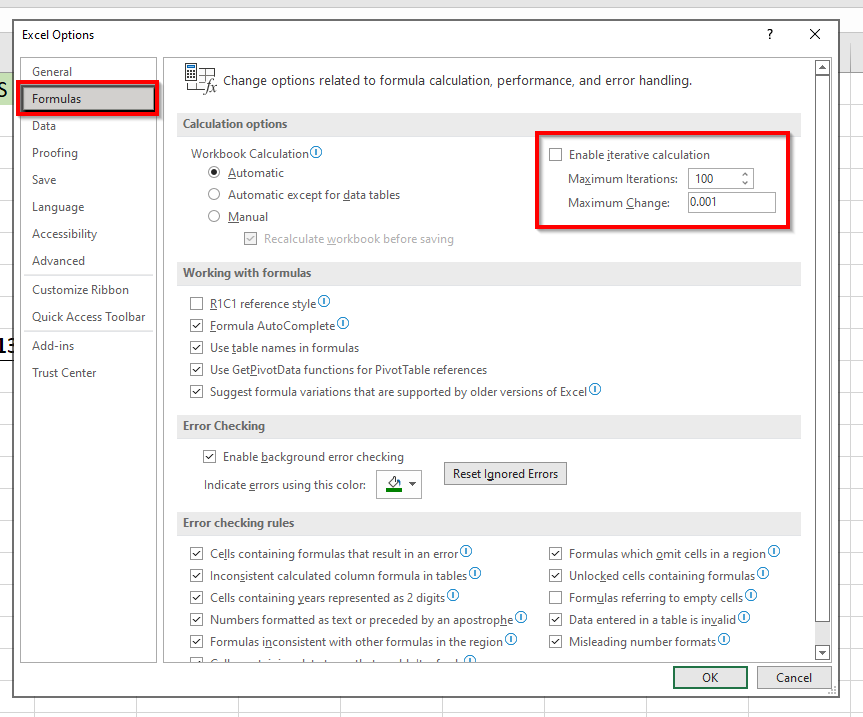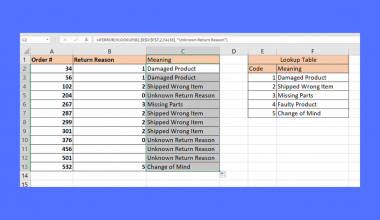# How to Find Circular References in Excel

This guide will explain how you can use Excel’s Error Checking feature to find circular reference errors in your spreadsheet.

Circular references refer to instances where an Excel formula refers back to its own cell.

Let’s take a look at a scenario that creates a circular reference in your spreadsheet.

If you type =A5 into cell A5, Excel will detect a circular reference. This works even if we add other functions or values to the formula. For instance, we could write `=A5 * 10` or `=A5<100`, which will still be considered a circular reference.

These Excel circular references can create an endless loop of calculations. To calculate the value of A5 * 10, Excel will look up the value of A5, which will prompt Excel to yet again retrieve the value of A5. Excel considers these circular references an error because they are impossible to evaluate.

Users may not intentionally create these errors but they are often created accidentally. In the example below, the formula in cell A8 is supposed to compute the `SUM` of the six numbers above it.The user accidentally included the current cell A8 in the range to sum up, causing a circular reference. Excel will helpfully return an error if this occurs.

In case other circular references appear in your sheet, Microsoft Excel includes a feature called Error Checking that can help find these circular references.

Though circular references are mostly accidental, there are some cases where using a circular reference could be a good solution for a particular problem. We’ll explore in-depth in the next section how we can allow and use a circular reference to create a static timestamp when a certain column is filled up.

Now that we know what circular references are, let’s look into how we can use the Error Checking function to find them and how we can use circular references for specific use cases.

## A Real Example of Using Circular References in Excel

Let’s take a look at a real example of a circular reference in a spreadsheet.

The table below has sales data for various items. Row 9 provides totals for the columns for Sales, Returns, and Pre-Orders. Using the Error Checking feature, we figure out that cell C9 has a circular reference that is causing it to return 0.The formula in column C was accidentally written to include cell C9 itself in the range to add. Changing the formula to have the correct range will allow it to return the right result.

Below is an example of a spreadsheet that intentionally uses circular references. We have nested IF statements that check if the cell is blank. Once it finds out that the keyword “yes” is written in column B, it writes down the current timestamp into the cell. Future evaluations will continue to write that timestamp until the value in column B changes.For this to work properly, we will have to allow for iterative calculations in spreadsheets. We will explore how to do this in the next section.

You can make your own copy of the tables above using the link attached below.

If you’re ready to try out circular references in Excel, head over to the next section to find out how to find them or use them to your benefit.

## How to Use Circular References in Excel

This section will be guiding you through each step needed to start handling circular references in Excel. You’ll learn how we can use the Error Checking feature to find circular references you may have missed. Later, you will look at how we can change our Excel software to allow iterative computations.

Follow these steps to start looking for circular references:

1. First, head over to the Formulas tab. Click on the Error Checking option to start Excel’s search for various errors. We can find any circular references once we hover over the Circular References option in the dropdown menu.2. The dropdown menu should return the results of our error search. In this example, we found a circular reference in cell C9.3. Lastly, users can manually adjust the cells that hold a circular reference. In the case below, we simply had to adjust the range from C2:C9 to C2:C8 to remove the circular reference.Excel disables iterative calculations by default but Excel has a setting that allows us to work with circular references.

1. By default, placing an iterative calculation returns a 0. In the example below, we placed the formula =I4+1 in cell I4 and it only returns 0.2. Access the Excel Options dialog found in the File tab. In the Formulas tab of the dialog box, look for the section labeled Calculation options. Here we have the option to enable iterative calculations and the maximum iterations allowed until the cell returns a value.In this example, we’ve set a maximum number of 100 iterations. Click on OK to change these settings.
3. When we place the formula in cell I4 again, it returns a value of 100. This is because we effectively added 1 to cell I4 100 times. If we edit any other cell, another hundred will be added to our cell.That’s all you need to remember to find circular references in Excel. This step-by-step guide shows how we can easily use the Error Checking feature to find cells that accidentally include an input of itself. You should also know how to start using iterative calculations to your advantage.

Circular references are just one example of an error you may encounter in Excel. With so many other Excel functions out there, you can surely find one that suits your use case.

Are you interested in learning more about what Excel can do? Stay notified of new guides like this by subscribing to our newsletter!### Get emails from us about Excel.

Our goal this year is to create lots of rich, bite-sized tutorials for Excel users like you. If you liked this one, you'd love what we are working on! Readers receive ✨ early access ✨ to new content.

##### You May Also Like## How to Get Rid of Scientific Notation in Excel

This guide will explain how to get rid of scientific notation in Excel using six easy and simple…## How to Find the Antilog of Values in Excel

This guide will help you find the antilog of a particular value in Excel. Antilogs or inverse logarithms…## How to Use ISERROR with VLOOKUP in Excel

This guide will explain how you can use the ISERROR function in Excel to catch and handle errors…## How to Calculate the Mean Absolute Deviation in Excel

This guide will explain how to calculate the mean absolute deviation in Excel. Excel is a popular tool…